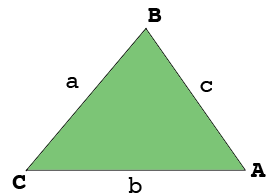SEARCH HOMEMath Central Quandaries & QueriesQuestion from Angelo: hi admin please help me answer this question. thank you! At a certain instant of time, the angle A of a triangle ABC is 60 degrees and increasing at the rate of 5degrees per second, the side AB is 10cm and increasing at the rate of 1cm per second, and side AC is 16cm and decreasing at the rate of 1/2 cm per second. Find the rate of change of side AB?Hi Angelo,

I drew your triangle, not to scale.The lengths of the sides are $a, b$ and $c$ as labeled, all in centimeters. Let $A$ be the measure of the angle at A in degrees and $C$ the measure of the angle at C in degrees. The side lengths and angle measures are all changing with time so they are all functions of time $t$ in seconds.

You know $b, c$ and $A$ at a particular time, say $t = t_0$ seconds and you also know the rate of change of these quantities at this time. You also Your question asks for the rate of change of the length of the side $AB$ which you already know. I think you are to find the rae of change of the length of the side $BC,$ that is $a$ at this time.

What you need is a mathematical expression involving $a, b, c$ and $A$ which you can differentiate with respect to $t.$ This will then give you an expression involving these three quantities and their derivatives. Five of these quantities you know at time $t_0$ seconds so you can solve for the sixth.

One problem remains, find a mathematical expression involving $a, b, c$ and $A.$Do you know one? Write back if you need more assistance.

Penny* Registered trade mark of Imperial Oil Limited. Used under license.Math Central is supported by the University of Regina and the Imperial Oil Foundation.